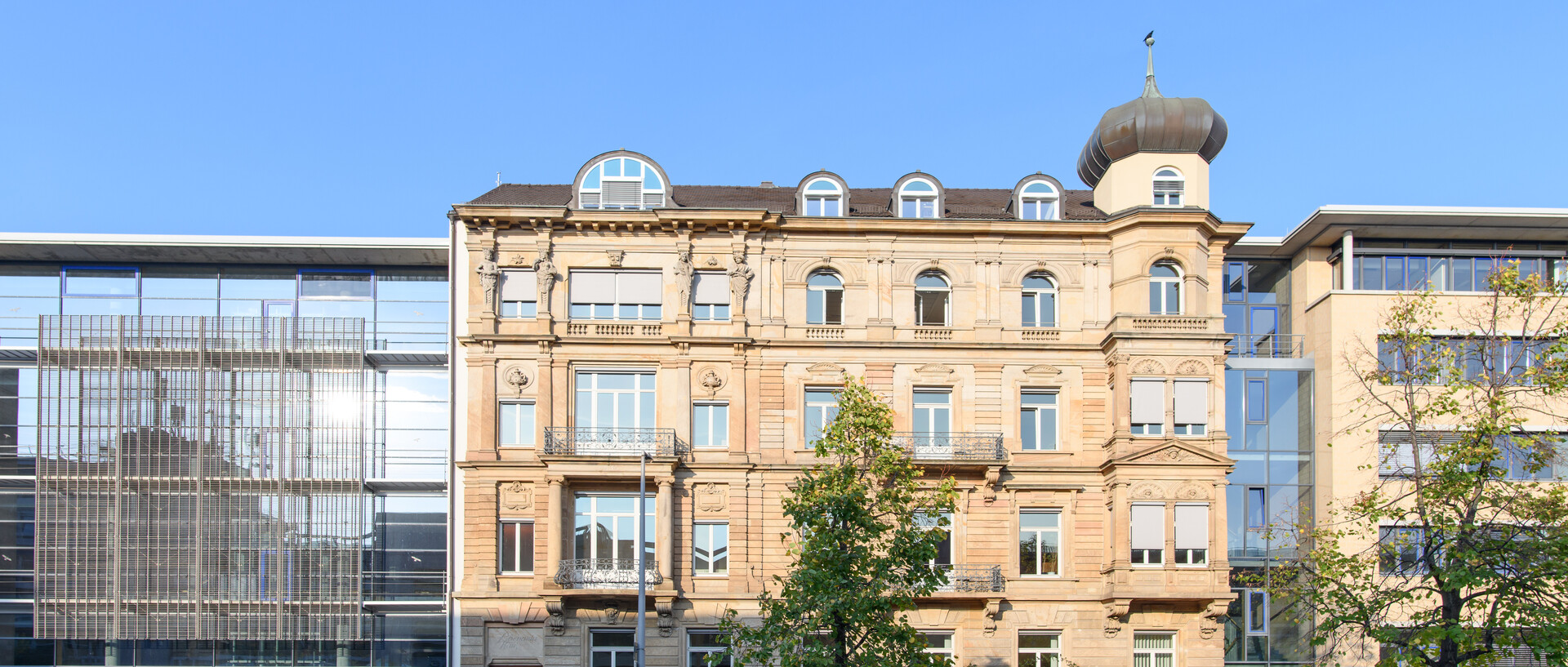# Numerics of ordinary differential equations

## Andreas Neuenkirch

Lecture with Exercise Class (MSc, 6 ECTS)

This lecture discusses standard concepts for solving ordinary differential equations (ODEs) numerically and introduces some applications.

Many problems in science and engineering can be modelled with the help of ODEs. Only few problems allow for analytical solutions, this motivates the study of numerical concepts.

Necessary prerequisite is the lecture “Dynamische Systeme (und Stabilität)”.

### Dates etc.

• First lecture: Monday, 4.9.2023,13:45 -- 15:15,  C 013 Hörsaal (A 5, 6 Bauteil C)
• First exercise class: Monday, 4.9.2023, 15:30 -- 17:00, C 013 Hörsaal (A 5, 6 Bauteil C)
• Admission to exam: 50 % of programming assignments (6 programming assignments in total); can be handed in in pairs
• Oral examinations; dates: tba

### Lectures

1. Ordinary differential equations (recap)
2. Consistency, stability and convergence in a nutshell
3. Consistency, stability and convergence: general theory
4. Explicit Runga-Kutta methods I
5. Explicit Runga-Kutta methods II
6. Stepsize control and stiffness
7. Stiffness and stability: the general problem
8. Consistent rational approximation and A-stability
9. Reversibility and isometries
10. Implicit Runga-Kutta methods I
11. Implicit Runga-Kutta methods II and recap on Gauss-quadrature
12. Collocation and B-stability
13. Multi-step methods
14. tba

### Literature

• Lectures Notes and Slides
• Iserles, A. (2008). A First Course in the Numerical Analysis of Differential Equations. Cambridge University Press.
• Deuflhard, P., Bornemann, F., Scientific Computing with Ordinary Differential Equations, Springer, 2002.
• Deuflhard, P., Bornemann, F., Numerische Mathematik II, de Gruyter, 2008.
• Moler, C. B., Numerical computing with MATLAB, SIAM, 2004.